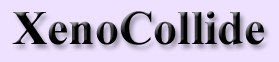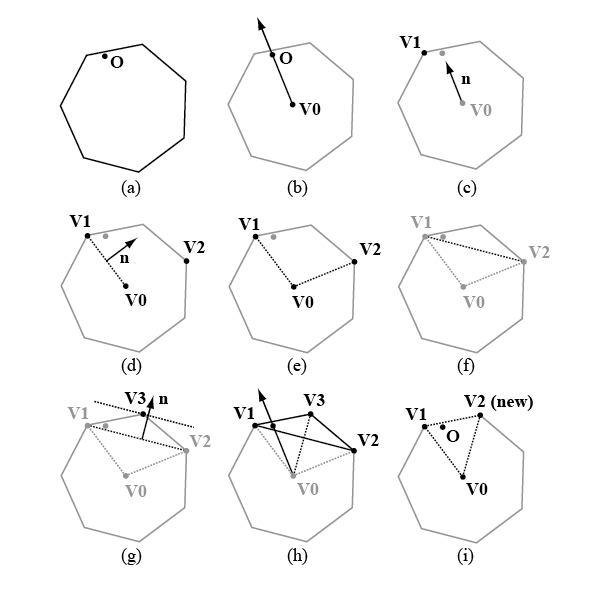Minkowski Portal Refinement in 2DFigure 1. An MPR algorithm in 2D

### Phase 1: Portal Discovery

Figure 1a. Here we see a convex shape, representing B–A, and the origin. Our objective is to determine if the origin lies within B–A, given only the support mapping of B–A to represent the surface.

Figure 1b. We obtain a point that we know lies somewhere deep within B–A. We can obtain such a point by subtracting any point deep within A from any point deep within B. The geometric centers of A and B are excellent choices.

Figure 1c. We construct a normal that originates at the interior point and points towards the origin. We then find the support point in the direction of this ray.

Figure 1d. We construct a ray that is perpendicular to the line between the support just discovered and the interior point. There are two choices for this ray, one for each side of the line segment. We choose the ray that lies on the same side of the segment as the origin. We use this ray to find a second support point on the surface of B–A.

Figure 1e. We now have three points, which form an angle. The origin lies somewhere within this angle.

Figure 1f. We create a line segment between the two support points. This line segment is called a portal, because the origin ray must pass through the line segment on its way to the origin.

### Phase 2: Portal Refinement

Figure 1f: If the origin lies on the same side of the portal as the interior point, then it lies within the dotted triangle, and must therefore lie within B–A. When this is the case, we terminate with a hit. In this example, the point lies on the outside of the portal, so the algorithm continues.

Figure 1g: We construct a normal perpendicular to the portal, pointing away from the interior. We use this normal to obtain a third support point on the surface of B–A. If the origin lies outside of the support line formed by the point and the normal, we know that the origin lies outside of B–A. In this case, the point lies on the inside of the support line, so the algorithm continues.

Figure 1h: The three support points form a triangle. We know the origin ray passes through the interior edge of this triangle, because it is a portal. It therefore must exit through one of the outer edges. To determine which edge it passes through, we construct a segment between the new support point and the interior point. If the origin lies on one side of the segment, the origin ray must pass through the outer edge that lies on the same side of the segment. If the origin lies on the other side, the origin ray must pass through the other outer edge.

Figure 1i: The outer edge that passes the test becomes the new portal, and we discard the unused support point. The algorithm continues from the beginning of phase 2, using the new portal. In the second iteration of our example, the origin lies on the inside of the new portal, so we will terminate with a hit.

Higher Dimensions

As demonstrated above, the 2D version of MPR constructs a ray that passes from an interior point through the origin. It then finds a portal that the ray passes through, and refines the portal by moving it closer and closer to the surface of the shape until the origin either lies inside the portal or outside a support line on the surface of the shape. The basic flow of MPR is the same in every dimension. In 3D, the portal becomes a triangle rather than a line segment. In 4D, the portal becomes a tetrahedron.